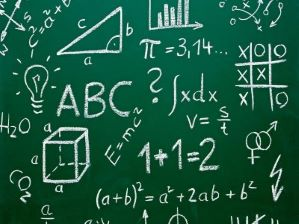# ALGEBRA I (Non-Interactive)\$ 15.00 \$ 5.00### Course Overview

Algebra I is an introductory course to provide early stage insight of one of the most important branch of Mathematics, Algebra. The students will learn what is physical meaning of an algebraic expression, how a longer algebraic expression can be simplified, what is utility of brackets in mathematics and why inequalities are important (with interpretation). At the end of this course, students will be able to translate a daily life problem to algebraic expression.

### Learning Objectives

• Ability to understand the relationship between Arithmetic and Algebra.
• Identify the differences and interpretation of variables, terms and expressions in Algebra.
• Use of simple operations on Algebraic expressions.
• Built the aptitude to simplify any expressions grouped with brackets.
• Demonstrate understanding of use of inequalities
• Create an understanding to express a simple real-world problem in mathematical notations.